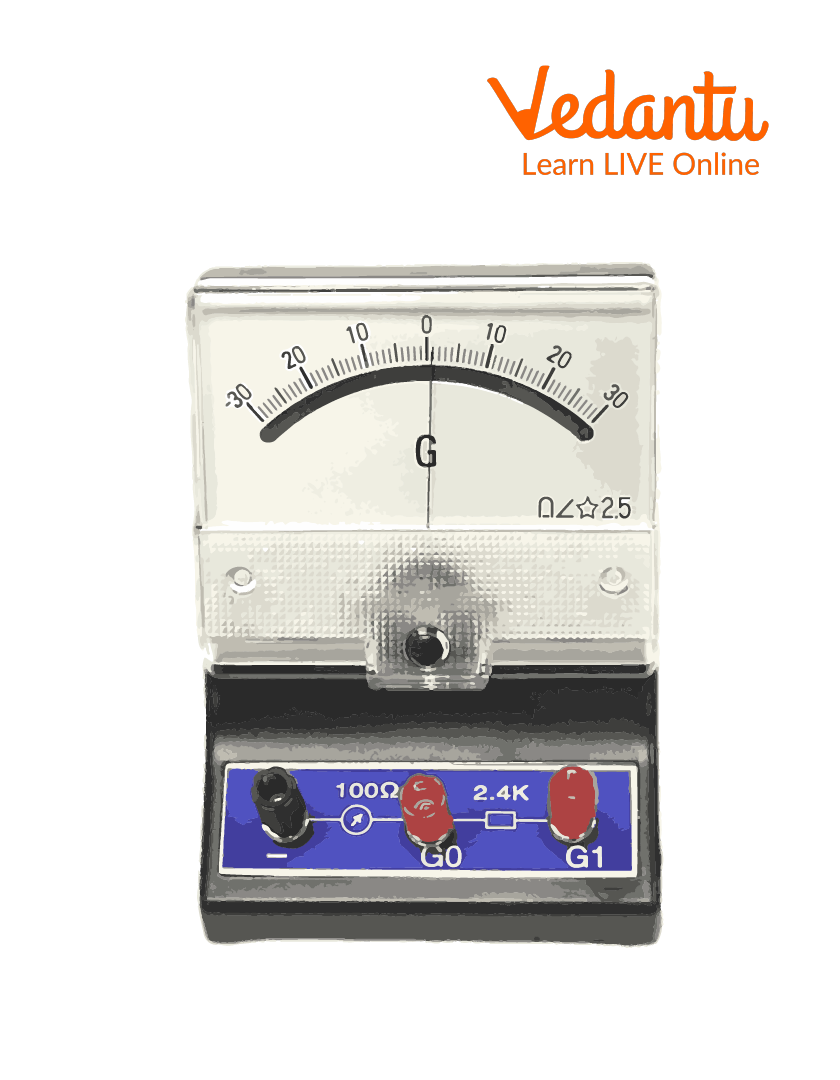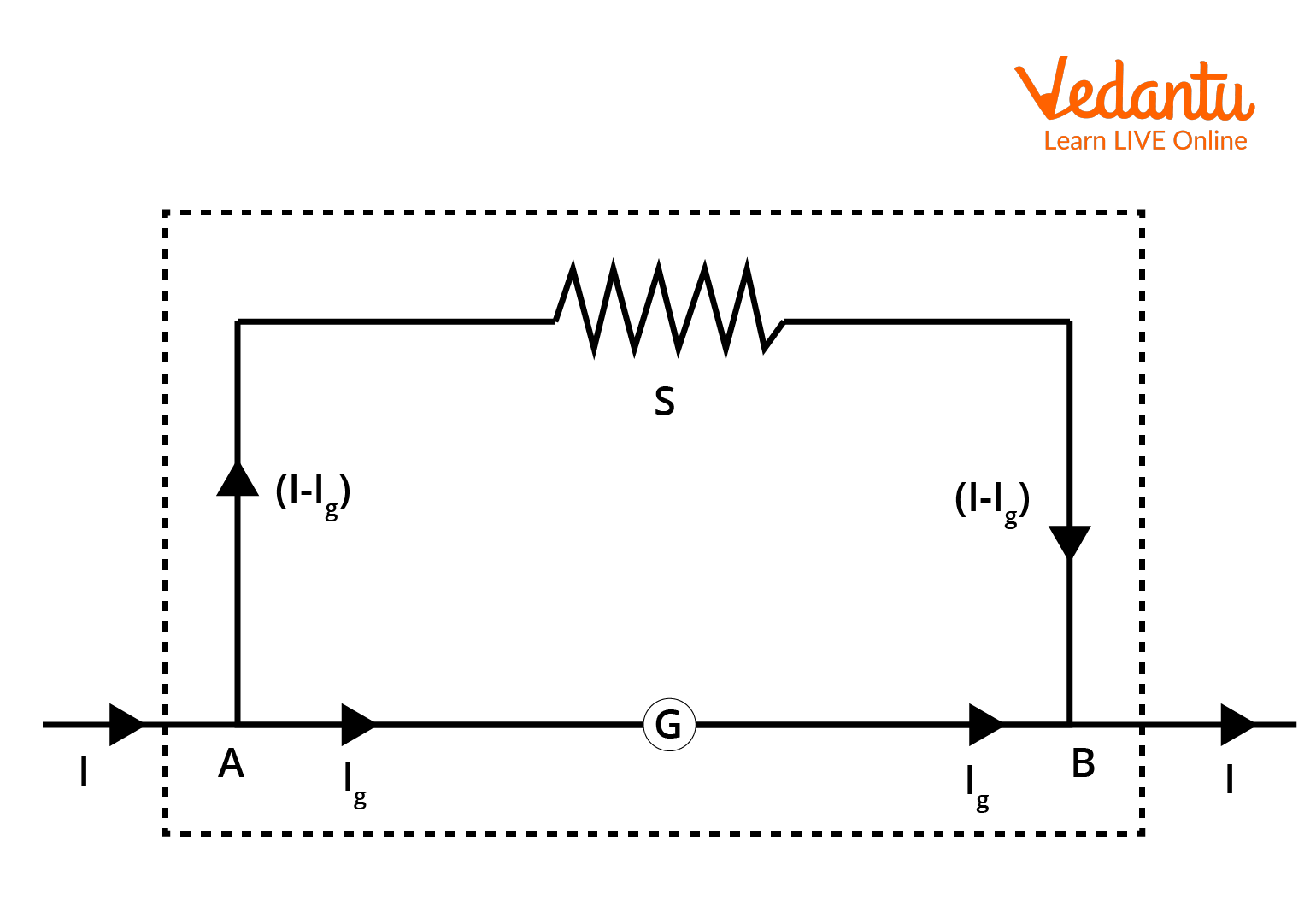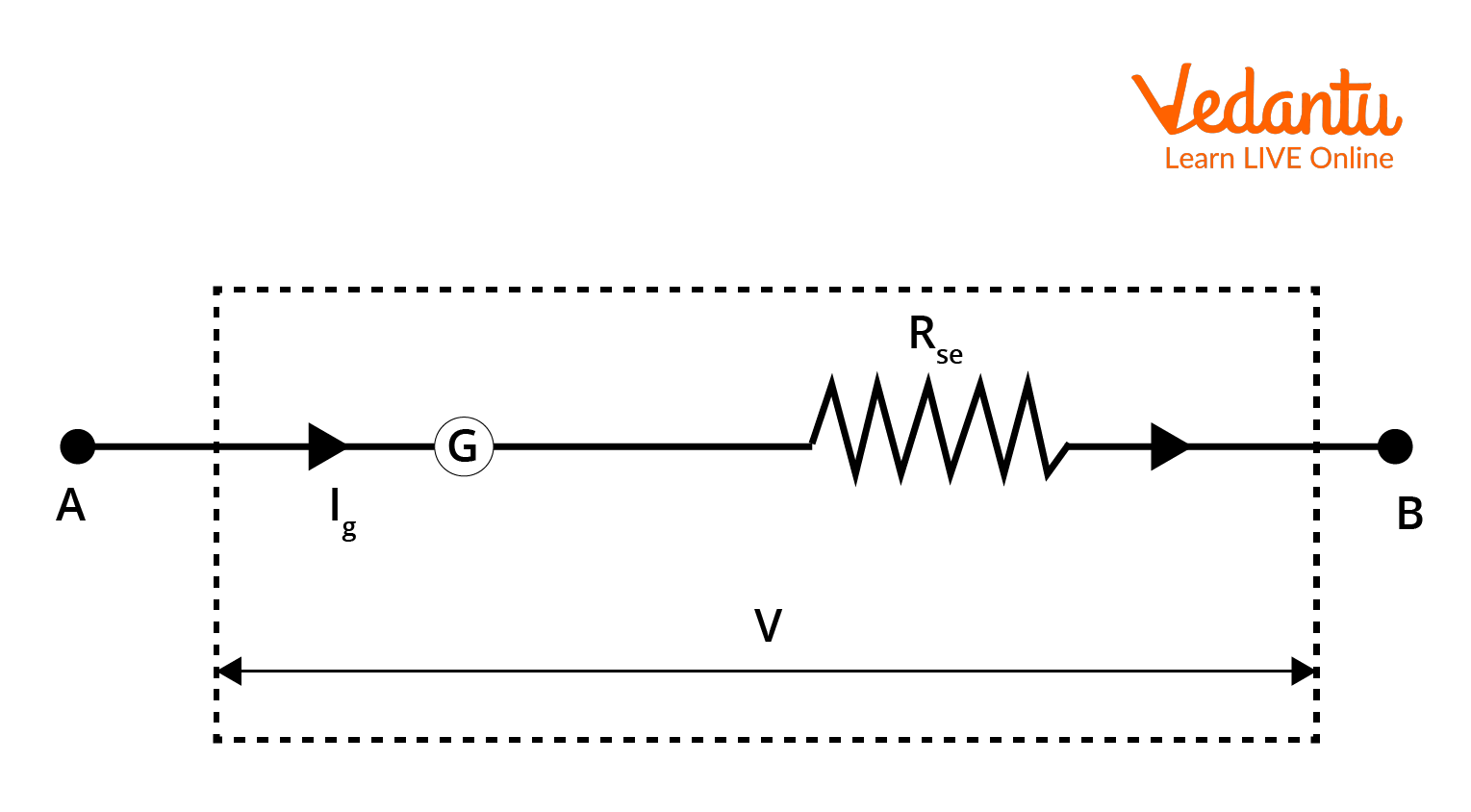Courses
Courses for Kids
Free study material
Free LIVE classes
More

# Conversion of Galvanometer to Ammeter and Voltmeter and Related Formulas| JEELIVE
Join Vedantu’s FREE Mastercalss

## Conversion of Galvanometer to Ammeter and Voltmeter

A galvanometer is an instrument that is used in the detection of small currents in an electric circuit. The conversion of galvanometer to ammeter and voltmeter respectively can easily be achieved by adding resistance in series or in shunt. But why do we require the conversion of galvanometer into ammeter and voltmeter? As mentioned earlier, a galvanometer is an instrument used to measure small electric currents. To measure high currents and high potential difference in an electric circuit, galvanometers are usually converted to ammeter or voltmeter depending on the required usage.

## What is a Galvanometer?

A galvanometer is a device or an instrument that is used to measure small currents in an electric circuit. It can be used as both ammeter and voltmeter by adding resistance to it in parallel or series. A galvanometer consists of a coil which is placed between the poles of a horseshoe magnet. When an electric current passes through this coil, the pointer of the galvanometer deflects. Most popular and frequently used galvanometer today is the D’Arsonal type.A Galvanometer

## Galvanometer to Ammeter Formula

The conversion of a galvanometer into an ammeter and a voltmeter is achieved by adding a resistor of a particular value in series or in parallel. A galvanometer can only measure small currents. Thus, for it to measure large currents, we convert it into an ammeter by connecting a resistor in parallel. Ammeter, as we know, is an instrument used to measure the value of current.  The ammeter must have low resistance, so that the current passing through it does not change. A low resistance value is connected in the shunt, so that the overall current passing through the ammeter does not change.Conversion of Galvanometer to Ammeter

The value of shunt S can be calculated using the circuit above. G is the resistance of the galvanometer, Ig is the current through the galvanometer, I is the total current through the circuit and (I-Ig) is the current passing through the shunt resistor (S).

Now, for a parallel circuit we know that the potential difference is equal. Thus, we get:

\begin{align} &{I_g} \times G=(I-{I_ g}) S \\ &\therefore S=\dfrac{{I_ g} G}{I-{I_ g}} \end{align}

or

$S=G\left(\frac{I_g}{I-I_g}\right)$

Further,

\begin{align} &S \times I=G \times I g+S \times I g \\ &\Rightarrow S \times I={I_g}(G+S) \\ &\Rightarrow I={I_g}\left(\dfrac{G+S}{S}\right) \\ &\text { or } \\ &\Rightarrow I={I_ g}\left(1+\dfrac{G}{S}\right) \end{align}

It can be further re-written as: $\Rightarrow {I_ g}=I\left(\dfrac{S}{G+S}\right)$

Now, if $\theta$ is assumed to be the deflection in the galvanometer, then it can be denoted as:

$\theta \propto {I_ g}$

$\theta \propto {I_ g}. m$, where $m=\dfrac{I}{I_g}$

$\theta \propto {I_ g} \cdot \dfrac{I}{I_g}$

$\theta \propto I$

Thus, deflection in the galvanometer is equal to the current passing through it. Additionally, the value of shunt resistance will be in milliohms.

## How to Convert a Galvanometer into a Voltmeter?

Since it is a very sensitive instrument, a galvanometer cannot measure high voltages or potential difference. Thus, to overcome this, we convert a galvanometer to a voltmeter.Conversion of Galvanometer to Voltmeter

Voltmeter, as we know, is used to measure the potential difference between any two points in an electric circuit. The current passing through the galvanometer must be equal to the total current in a circuit, as the difference will cause a change in the potential difference. Thus, we connect a high resistance in series with a galvanometer.

## Galvanometer to Voltmeter Formula

Assuming Ig as the value of galvanometer, V is the potential difference or emf between point A and B, Rse is the series resistance connected to galvanometer and $\theta$ is the deflection in the galvanometer. Also, Rg is the resistance of the galvanometer and Vm is the voltage across Rse.

As it is a series circuit, I=Ig

$I_g=\dfrac{V}{R_{se}+R_g}$

Thus, $I_g\propto V$, which means that the deflection in the galvanometer depends on the potential difference across any two points in the circuit.

Further,

\begin{align} &\dfrac{V}{R_g}=\dfrac{(V-V_m)}{R_{se}} \\ &\dfrac{R_{se}}{R_g}=\dfrac{V-V_m}{V} \\ &\therefore \dfrac{V}{V_m}=\left(1+\dfrac{R_{s e}}{R_g}\right) \\ &\Lambda l s o, m=\dfrac{V}{V_m} \\ &\therefore m-1=\dfrac{R_{s e}}{R_ g} \end{align}

The value of Rse or series resistor will be in kiloohms ($k\Omega$). Also, the scale of the galvanometer will now be in volts and its range will depend on the value of resistance connected in series.

## Conclusion

The article presents a thorough overview of how a galvanometer is converted to an ammeter and a voltmeter respectively. Being a sensitive device, a galvanometer can only measure feeble currents in the circuit. Thus, to extend its range, we use resistors in series and in parallel, to control the flow of current through the galvanometer. Also, the branches of resistors to be connected depends on how much the range of ammeter or voltmeter is to be increased. To increase the range of ammeter n times, the value of shunt resistor to be used is $S=\dfrac{G}{n-1}$, whereas for voltmeter, it will be $R=(n-1) \cdot G$.

Last updated date: 18th Sep 2023
Total views: 132k
Views today: 4.31k

## FAQs on Conversion of Galvanometer to Ammeter and Voltmeter and Related Formulas| JEE

1. What are D'Arsonval and moving magnet galvanometers?

D'Arsonval galvanometer is also known as moving coil galvanometer. Moving coil galvanometer is a very sensitive instrument used to measure feeble currents of the order of $10^{-9}$. It consists of a coil, and the deflection in this galvanometer is dependent on the amount of current passing through it.

Moving magnet on the other hand is a type of galvanometer that is dependent on the movement of a small magnet. The magnet is essentially a permanent magnet placed in a magnet field, and its movement is dependent on the amount of current passing through it.

2. What is the material used for the coil of the galvanometer?

The coil in a galvanometer is a current carrying part of it. A thin copper insulated wire is used as a coil in a galvanometer. The coil used may be rectangular or circular, and is wound with a number of turns. For example, modern galvanometers like D'Arsonval are constructed with a small pivoting coil of wire. It is called a spindle and is placed in a magnetic field. The coil is further attached to a pointer that traverses over a calibrated scale to provide the measured value.